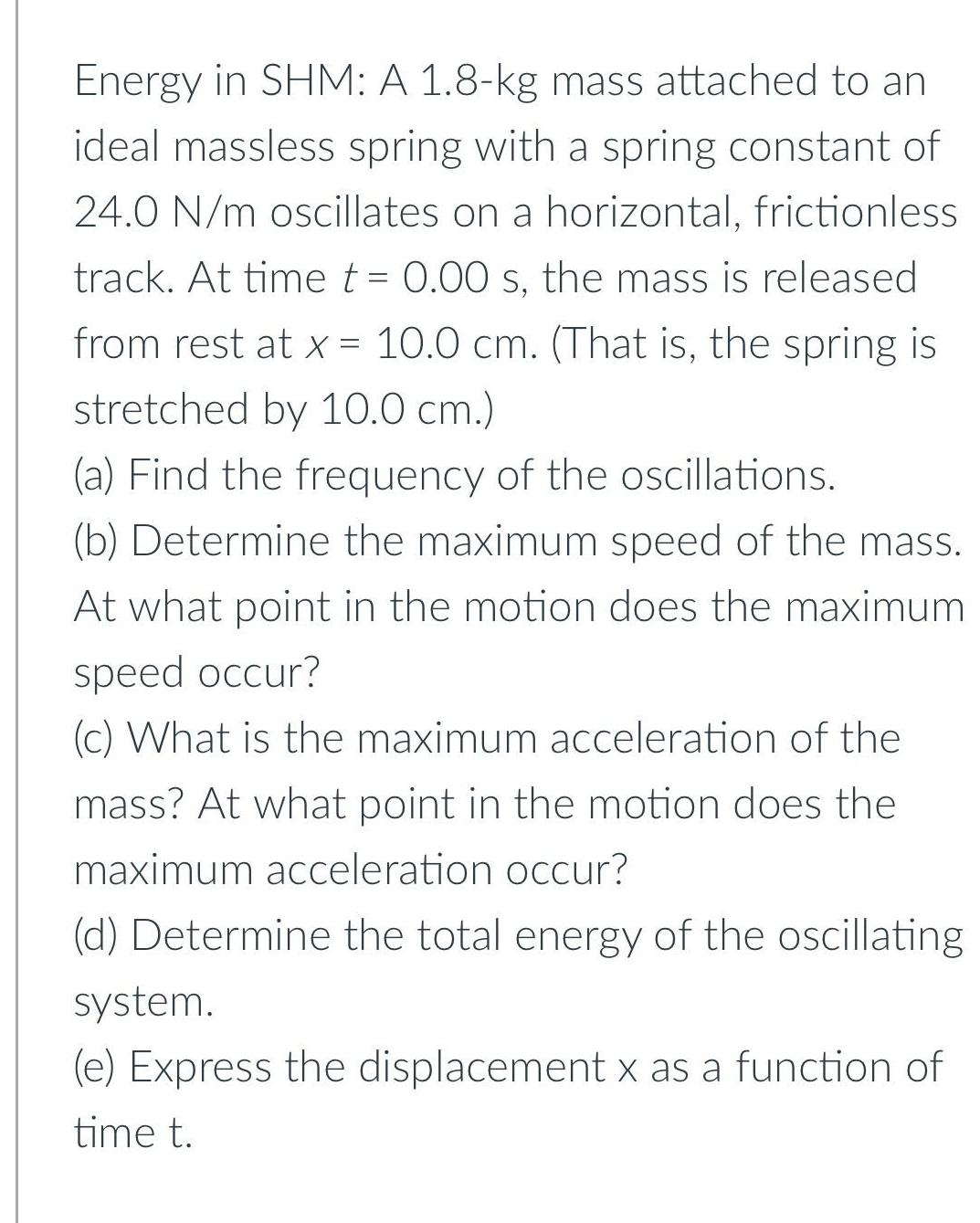Question:

# Energy in SHM: A 1.8-kg mass attached to an ideal massless

Last updated: 8/2/2022Energy in SHM: A 1.8-kg mass attached to an ideal massless spring with a spring constant of 24.0 N/m oscillates on a horizontal, frictionless track. At time t = 0.00 s, the mass is released from rest at x = 10.0 cm. (That is, the spring is stretched by 10.0 cm.) (a) Find the frequency of the oscillations. (b) Determine the maximum speed of the mass. At what point in the motion does the maximum speed occur? (c) What is the maximum acceleration of the mass? At what point in the motion does the maximum acceleration occur? (d) Determine the total energy of the oscillating system. (e) Express the displacement x as a function of time t.